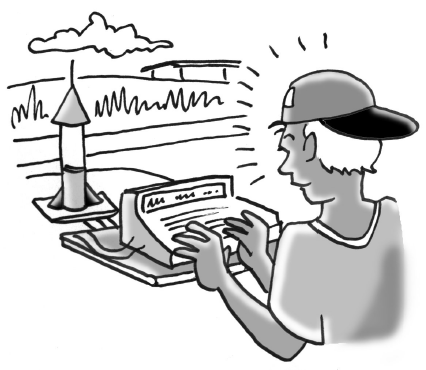### Home > CCA > Chapter 10 > Lesson 10.2.5 > Problem10-86

10-86.Chad is entering a rocket competition. He needs to program his rocket so that when it is launched from the ground, it lands $20$ feet away. In order to qualify, it must be $100$ feet off the ground at its highest point. What equation should he program into his rocket launcher to win? Let $x$ represent the distance from the launch pad in feet and $y$ represent the height of the rocket in feet. Draw a sketch of the rocket’s path.

Find the $x$-intercepts.

Substitute the $x$-intercept values into the equation formula. $y=a(x-\underline{\hspace{0.5cm}}\ )(x-\underline{\hspace{0.5cm}}\ )$

Find the vertex.

Substitute the vertex into the equation you found so far and solve for $a$.

Divide both sides by $-100$.

$(0,0)$ and $(20,0)$

$y=a(x+0)(x-20)$
$y=a(x)(x-20)$

$(10,100)$

$100=a(10)(10-20)$
$100=a(10)(-10)$
$100=a(-100)$

$-1=a$

The equation is $y=(-1)(x)(x-20)$ or $y=-x^2+20x$.

Program the equation you found above into box 5 below to see the rocket's path.
Click on the link at right for the full eTool version: CCA 10-86 HW eTool (Desmos)